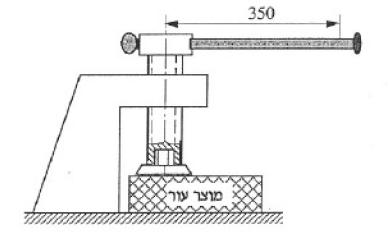# Power screw question - Help

dislect
Hi all

1. The pressure device described in the pic needs to apply a force of 10kN on the 'square product' below in order to work. The worker can apply 100N force while the 'arm' is 350mm.
Assuming the friction (mew) can change from 0.05 to 0.15 and the thread is square (alpha=0) design a screw for minimum turns.
2. Sy=210 MPa, n (safety coefficient)=3, N=1 (number of 'starts' is it called?), find the screw max length against collisionI'm still in 1, im not sure how to look at it. can someone give me a head start in this question? how do you create an equation for minimum turns?

thanks

JaredJames
Really speaking, this should be in the homework section. But, here goes:

Well the minimum number of turns will be until it contacts the item you are applying the force to. Until that point, there is zero force being applied.

Have you calculated how much torque the workers available force translates to?

dislect
i thought i published it in the HW section :\ can someone move it?
isnt the momentum 0.350*100 ?
i thought that min turns means the numer of turns untill the pressure build up to 10KN

JaredJames
Sorry, as per the question, yes minimum turns is until the pressure reaches 10KN.